# Precession

﻿
Precession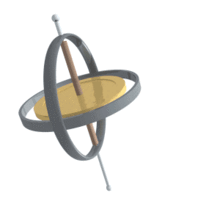Precession of a gyroscope

Precession is a change in the orientation of the rotation axis of a rotating body. It can be defined as a change in direction of the rotation axis in which the second Euler angle (nutation) is constant. In physics, there are two types of precession: torque-free and torque-induced.

In astronomy, "precession" refers to any of several slow changes in an astronomical body's rotational or orbital parameters, and especially to the Earth's precession of the equinoxes. See Precession (astronomy).

## Torque-free

Torque-free precession occurs when the axis of rotation differs slightly from an axis about which the object can rotate stably: a maximum or minimum principal axis. Poinsot's construction is an elegant geometrical method for visualizing the torque-free motion of a rotating rigid body. For example, when a plate is thrown, the plate may have some rotation around an axis that is not its axis of symmetry. This occurs because the angular momentum (L) is constant in absence of torques. Therefore, it will have to be constant in the external reference frame, but the moment of inertia tensor (I) is non-constant in this frame because of the lack of symmetry. Therefore, the spin angular velocity vector (ωs) about the spin axis will have to evolve in time so that the matrix product L = Iωs remains constant.

When an object is not perfectly solid, internal vortices will tend to damp torque-free precession, and the rotation axis will align itself with one of the inertia axes of the body.

The torque-free precession rate of an object with an axis of symmetry, such as a disk, spinning about an axis not aligned with that axis of symmetry can be calculated as follows:$\boldsymbol\omega_p = (I_s/I_p) \omega_s (1-\cos(\alpha))$

where$\boldsymbol\omega_p$ is the precession rate,$\boldsymbol\omega_s$ is the spin rate about the axis of symmetry,$\boldsymbol\alpha$ is the angle between the axis of symmetry and the axis about which it precesses,$\boldsymbol I_s$ is the moment of inertia about the axis of symmetry, and$\boldsymbol I_p$ is moment of inertia about either of the other two perpendicular principal axes. They should be the same, due to the symmetry of the disk.

For a generic solid object without any axis of symmetry, the evolution of the object's orientation, represented (for example) by a rotation matrix$\boldsymbol R$ that transforms internal to external coordinates, may be numerically simulated. Given the object's fixed internal moment of inertia tensor$\boldsymbol I_0$ and fixed external angular momentum$\boldsymbol L$, the instantaneous angular velocity is$\boldsymbol\omega(\boldsymbol R) = \boldsymbol R \boldsymbol I_0^{-1} \boldsymbol R ^T \boldsymbol L$. Precession occurs by repeatedly recalculating$\boldsymbol \omega$ and applying a small rotation vector$\boldsymbol \omega dt$ for the short time dt, e.g.$\boldsymbol R_{new} = \exp([\boldsymbol\omega(\boldsymbol R_{old})]_{\times} dt) \boldsymbol R_{old}$ for the skew-symmetric matrix$[\boldsymbol\omega]_{\times}$. The errors induced by finite time steps tend to increase the rotational kinetic energy,$E(\boldsymbol R) = \boldsymbol \omega(\boldsymbol R) \cdot \boldsymbol L / 2$; this unphysical tendency can be counter-acted by repeatedly applying a small rotation vector$\boldsymbol v$ perpendicular to both$\boldsymbol \omega$ and$\boldsymbol L$, noting that$E(\exp([\boldsymbol v]_{\times}) \boldsymbol R) \approx E(\boldsymbol R) + (\boldsymbol \omega(\boldsymbol R) \times \boldsymbol L) \cdot \boldsymbol v$.

Another type of torque-free precession can occur when there are multiple reference frames at work. For example, the earth is subject to local torque induced precession due to the gravity of the sun and moon acting upon the earth’s axis, but at the same time the solar system is moving around the galactic center. As a consequence, an accurate measurement of the earth’s axial reorientation relative to objects outside the frame of the moving galaxy (such as distant quasars commonly used as precession measurement reference points) must account for a minor amount of non-local torque-free precession, due to the solar system’s motion.

## Torque-induced

Torque-induced precession (gyroscopic precession) is the phenomenon in which the axis of a spinning object (e.g., a part of a gyroscope) "wobbles" when a torque is applied to it. The phenomenon is commonly seen in a spinning toy top, but all rotating objects can undergo precession. If the speed of the rotation and the magnitude of the torque are constant, the axis will describe a cone, its movement at any instant being at right angles to the direction of the torque. In the case of a toy top, if the axis is not perfectly vertical, the torque is applied by the force of gravity tending to tip it over.The response of a rotating system to an applied torque. When the device swivels, and some roll is added, the wheel tends to pitch.

The device depicted on the right here is gimbal mounted. From inside to outside there are three axes of rotation: the hub of the wheel, the gimbal axis, and the vertical pivot.

To distinguish between the two horizontal axes, rotation around the wheel hub will be called 'spinning', and rotation around the gimbal axis will be called 'pitching.' Rotation around the vertical pivot axis is called 'rotation'.

First, imagine that the entire device is rotating around the (vertical) pivot axis. Then, spinning of the wheel (around the wheelhub) is added. Imagine the gimbal axis to be locked, so that the wheel cannot pitch. The gimbal axis has sensors, that measure whether there is a torque around the gimbal axis.

In the picture, a section of the wheel has been named dm1. At the depicted moment in time, section dm1 is at the perimeter of the rotating motion around the (vertical) pivot axis. Section dm1, therefore, has a lot of angular rotating velocity with respect to the rotation around the pivot axis, and as dm1 is forced closer to the pivot axis of the rotation (by the wheel spinning further), due to the Coriolis effect dm1 tends to move in the direction of the top-left arrow in the diagram (shown at 45°) in the direction of rotation around the pivot axis. Section dm2 of the wheel starts out at the vertical pivot axis, and thus initially has zero angular rotating velocity with respect to the rotation around the pivot axis, before the wheel spins further. A force (again, a Coriolis force) would be required to increase section dm2's velocity up to the angular rotating velocity at the perimeter of the rotating motion around the pivot axis. If that force is not provided, then section dm2's inertia will make it move in the direction of the top-right arrow. Note that both arrows point in the same direction.

The same reasoning applies for the bottom half of the wheel, but there the arrows point in the opposite direction to that of the top arrows. Combined over the entire wheel, there is a torque around the gimbal axis when some spinning is added to rotation around a vertical axis.

It is important to note that the torque around the gimbal axis arises without any delay; the response is instantaneous.

In the discussion above, the setup was kept unchanging by preventing pitching around the gimbal axis. In the case of a spinning toy top, when the spinning top starts tilting, gravity exerts a torque. However, instead of rolling over, the spinning top just pitches a little. This pitching motion reorients the spinning top with respect to the torque that is being exerted. The result is that the torque exerted by gravity - via the pitching motion - elicits gyroscopic precession (which in turn yields a counter torque against the gravity torque) rather than causing the spinning top to fall to its side.

Precession or gyroscopic considerations have an effect on bicycle performance at high speed. Precession is also the mechanism behind gyrocompasses.

Gyroscopic precession also plays a large role in the flight controls on helicopters. Since the driving force behind helicopters is the rotor disk (which rotates), gyroscopic precession comes into play. If the rotor disk is to be tilted forward (to gain forward velocity), its rotation requires that the downward net force on the blade be applied roughly 90 degrees (depending on blade configuration) before, or when the blade is to one side of the pilot and rotating forward.

To ensure the pilot's inputs are correct, the aircraft has corrective linkages that vary the blade pitch in advance of the blade's position relative to the swashplate. Although the swashplate moves in the intuitively correct direction, the blade pitch links are arranged to transmit the pitch in advance of the blade's position.

### Classical (Newtonian)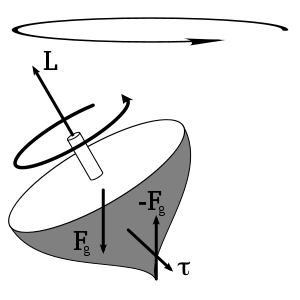The torque caused by the two opposing forces Fg and -Fg causes a change in the angular momentum L in the direction of that torque. This causes the top to precess.

Precession is the result of the angular velocity of rotation and the angular velocity produced by the torque. It is an angular velocity about a line that makes an angle with the permanent rotation axis, and this angle lies in a plane at right angles to the plane of the couple producing the torque. The permanent axis must turn towards this line, since the body cannot continue to rotate about any line that is not a principal axis of maximum moment of inertia; that is, the permanent axis turns in a direction at right angles to that in which the torque might be expected to turn it. If the rotating body is symmetrical and its motion unconstrained, and, if the torque on the spin axis is at right angles to that axis, the axis of precession will be perpendicular to both the spin axis and torque axis.

Under these circumstances the angular velocity of precession is given by:$\boldsymbol\omega_p = \frac{\ mgr}{I_s\boldsymbol\omega_s}$

In which Is is the moment of inertia,$\boldsymbol\omega_s$ is the angular velocity of spin about the spin axis, and m*g*r are the force and radius that comes from the torque. The torque vector originates at the center of mass. Using$\boldsymbol\omega$ =$\frac{2\pi}{T}$, we find that the period of precession is given by:$T_p = \frac{4\pi^2I_s}{\ mgrT_s}$

In which Is is the moment of inertia, Ts is the period of spin about the spin axis, and$\boldsymbol\tau$ is the torque. In general, the problem is more complicated than this, however.

### Relativistic

The special and general theories of relativity give three types of corrections to the Newtonian precession, of a gyroscope near a large mass such as the earth, described above. They are:

• Thomas precession a special relativistic correction accounting for the observer's being in a rotating non-inertial frame.
• de Sitter precession a general relativistic correction accounting for the schwarzschild metric of curved space near a large non-rotating mass.
• Lense-Thirring precession a general relativistic correction accounting for the frame dragging by the Kerr metric of curved space near a large rotating mass.

## Astronomy

In astronomy, precession refers to any of several gravity-induced, slow and continuous changes in an astronomical body's rotational axis or orbital path.

### Axial precession (precession of the equinoxes)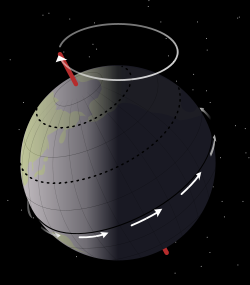Precessional movement.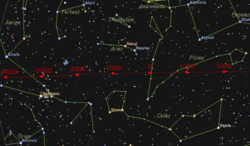Precession of the equinox in relation to the distant stars

Axial precession is the movement of the rotational axis of an astronomical body, whereby the axis slowly traces out a cone. In the case of Earth, this type of precession is also known as the precession of the equinoxes or precession of the equator. Earth goes through one such complete precessional cycle in a period of approximately 26,000 years, during which the positions of stars as measured in the equatorial coordinate system will slowly change; the change is due to the change of the coordinates. Over this cycle, Earth's north axial pole moves from where it is now, within 1° of Polaris, in a circle around the ecliptic pole, with an angular radius of about 23.5 degrees (or approximately 23 degrees 27 arcminutes ). The shift is 1 degree in 72 years, where the angle is taken from the observer, not from the center of the circle.

Hipparchus is the earliest known astronomer to recognize and assess the precession of the equinoxes at almost 1º per century (which is not far from the actual value for antiquity, 1.38º). The Precession (axial rotation) was later explained by Newtonian physics. Being an oblate spheroid, the Earth has a nonspherical shape, bulging outward at the equator. The gravitational tidal forces of the Moon and Sun apply torque as they attempt to pull the equatorial bulge into the plane of the ecliptic. The portion of the precession due to the combined action of the Sun and the Moon is called lunisolar precession.

### Precession of the ecliptic

The inclination of Earth's orbit drifts up and down. Relative to its present orbit this drift has a period of about 70,000 years. Relative to the invariable plane it has a 100,000-year period. The invariable plane represents the angular momentum of the solar system, and is approximately the orbital plane of Jupiter.

### Perihelion precession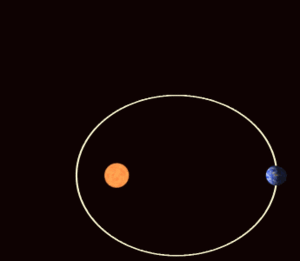Planets revolving the Sun follow elliptical (oval) orbits that rotate gradually over time (apsidal precession). The eccentricity of this ellipse is exaggerated for visualization. Most orbits in the Solar System have a much smaller eccentricity, making them nearly circular.

The orbit of a planet around the Sun is not really an ellipse but a flower-petal shape because the major axis of each planet's elliptical orbit also precesses within its orbital plane, partly in response to perturbations in the form of the changing gravitational forces exerted by other planets. This is called perihelion precession or apsidal precession.

Discrepancies between the observed perihelion precession rate of the planet Mercury and that predicted by classical mechanics were prominent among the forms of experimental evidence leading to the acceptance of Einstein's Theory of Relativity (in particular, his General Theory of Relativity), which accurately predicted the anomalies.

These periodic changes of Earth's orbital parameters, combined with the precession of the equinoxes and of the inclination of the Earth's axis on its orbit, are an important part of the astronomical theory of ice ages. See also nodal precession. For precession of the lunar orbit see lunar precession.

Wikimedia Foundation. 2010.

### Look at other dictionaries:

• Precession — Précession Précession d un gyroscope La précession est le nom donné au changement graduel d orientation de l axe de rotation d un objet ou, de façon plus générale, d un vecteur sous l action de l environnement, par exemple, quand un couple lui… …   Wikipédia en Français

• Précession — d un gyroscope La précession est le nom donné au changement graduel d orientation de l axe de rotation d un objet ou, de façon plus générale, d un vecteur sous l action de l environnement, par exemple, quand un couple lui est appliqué. Ce… …   Wikipédia en Français

• précession — [ presesjɔ̃ ] n. f. • 1690; lat. tardif præcessio ♦ Mouvement de rotation autour d un axe fixe, de l axe d un gyroscope. ♢ Mouvement analogue de l axe de rotation terrestre autour d une position moyenne de cet axe. Vitesse de précession : vitesse …   Encyclopédie Universelle

• Precession — Pre*ces sion, n. [L. praecedere, praecessum, to go before: cf. F. pr[ e]cession. See {Precede}.] The act of going before, or forward. [1913 Webster] {Lunisolar precession}. (Astron.) See under {Lunisolar}. {Planetary precession}, that part of the …   The Collaborative International Dictionary of English

• precession — [prē sesh′ən, prisesh′ən] n. [ME < LL praecessio < L praecedere, to PRECEDE] 1. the act of preceding; precedence 2. Astronomy short for PRECESSION OF THE EQUINOXES 3. Mech. an effect exhibited by a spinning body, as a top, when an applied… …   English World dictionary

• precession — precession. См. прецессия. (Источник: «Англо русский толковый словарь генетических терминов». Арефьев В.А., Лисовенко Л.А., Москва: Изд во ВНИРО, 1995 г.) …   Молекулярная биология и генетика. Толковый словарь.

• precession — (n.) 1590s, from L.L. praecissionem (nom. praecissio) a coming before, from pp. stem of L. praecedere (see PRECEDE (Cf. precede)). Originally used of calculations of the equinoxes, which come slightly earlier each year …   Etymology dictionary

• precession — ► NOUN 1) the slow movement of the axis of a spinning body around another axis. 2) Astronomy the slow retrograde motion of the equinoctial points along the ecliptic, resulting in the earlier occurrence of equinoxes each year. DERIVATIVES precess… …   English terms dictionary

• precession — precessional, adj. /pree sesh euhn/, n. 1. the act or fact of preceding; precedence. 2. Mech. the motion of the rotation axis of a rigid body, as a spinning top, when a disturbing torque is applied while the body is rotating such that the… …   Universalium

• précession — (pré sè ssion) s. f. Terme d astronomie. Précession des équinoxes, mouvement rétrograde des points équinoxiaux. •   Il [Newton] trouve par une méthode dont on ne saurait trop admirer la finesse, que la précession annuelle des équinoxes doit être… …   Dictionnaire de la Langue Française d'Émile Littré